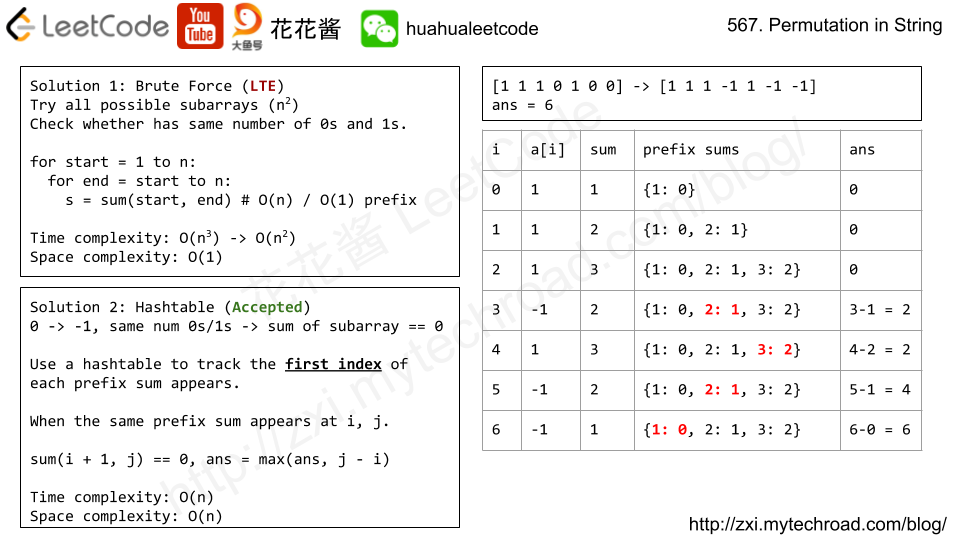Amazon

Apple

2020-05-26

## 525. Contiguous Array

### Question:

Given a binary array, find the maximum length of a contiguous subarray with equal number of 0 and 1.

#### Example 1:

``````Input: [0,1]
Output: 2
Explanation: [0, 1] is the longest contiguous subarray with equal number of 0 and 1.
``````

#### Example 2:

``````Input: [0,1,0]
Output: 2
Explanation: [0, 1] (or [1, 0]) is a longest contiguous subarray with equal number of 0 and 1.
``````

Note: The length of the given binary array will not exceed 50,000.

### Solution:

Using hash map to record the first sum value and its corresponding index. When finding the same sum value from the hash table, it means the gap between two index has the equal number of 0 and 1. To make sure the sum works in this case, we need to change all `0` to `-1`.

The following picture demo borrowed from Huahua.``````class Solution {
public int findMaxLength(int[] nums) {
if (nums == null || nums.length == 0) return 0;
HashMap<Integer, Integer> map = new HashMap<Integer, Integer>();
int result = 0;
int sum = 0;

map.put(0, -1);
for (int i = 0; i < nums.length; i++) {
int curr = nums[i];
sum += curr == 0 ? -1 : 1;
if (map.containsKey(sum)) {
result = Math.max(result, i - map.get(sum));
} else {
map.put(sum, i);
}
}

return result;
}
}
``````